Abstract: In this paper we are concerned with the Hochstadt-Lieberman uniqueness theorem which states that, when the potential is known a priori on [0, 1/2], the full Dirichlet-Dirichlet spectrum of a Sturm-Liouville problem defined on the interval [0, 1] uniquely determines its potential. We shall give a new method for reconstructing the potential for this problem in terms of the Mittag-Leffler decomposition Theorem of meromorphic functions associated with the solution of Sturm-Liouville equantions. We also give a necessary and sufficient condition for the existence of the solution.

1. 引言

$Lu=-{u}^{″}+qu$ (1)

$u\left(0\right)=0=u\left(1\right)$ , (2)

Martinyuk及Pivoarchik  曾对以上唯一性定理给出了重构势函数的方法。本文的目的是对Hochstadt-Lieberman唯一性定理提供一种新的重构势函数的方法。通过应用Mittag-Leffler展开定理，将“较大的”全纯函数分解为两个“较小的”全纯函数，此分解为我们更好地使用Levin-Lyubarski插值公式重构全纯函数 ${u}_{-}\left(1/2,\lambda \right)$${{u}^{\prime }}_{-}\left(1/2,\lambda \right)$ 提供了环境。此外，该重构方法亦给出了该问题的解存在且唯一的充要条件。

2. 势函数的重构

${u}_{-}\left(x,\lambda \right)$ 为方程(1)满足初始条件 ${u}_{-}\left(0\right)=0$${{u}^{\prime }}_{-}\left(0\right)=1$ 的解。由文  可得：

$\begin{array}{c}{u}_{-}\left(x,\lambda \right)=\frac{\mathrm{sin}\lambda x}{\lambda }+{\int }_{0}^{x}K\left(x,t\right)\frac{\mathrm{sin}\lambda t}{\lambda }\text{d}t\\ =\frac{\mathrm{sin}\lambda x}{\lambda }-K\left(x,x\right)\frac{\mathrm{cos}\lambda x}{{\lambda }^{2}}+{\int }_{0}^{x}{K}_{t}\left(x,t\right)\frac{\mathrm{cos}\lambda x}{{\lambda }^{2}}\text{d}t\end{array}$ , (3)

$K\left(x,t\right)=\stackrel{˜}{K}\left(x,t\right)-\stackrel{˜}{K}\left(x,-t\right)$ , ${K}_{t}\left(x,t\right)=\frac{\partial K\left(x,t\right)}{\partial t}$ ,

$\stackrel{˜}{K}\left(x,t\right)$ 满足以下积分方程：

$\stackrel{˜}{K}\left(x,t\right)=\frac{1}{2}{\int }_{0}^{\frac{x+t}{2}}q\left(s\right)\text{ds}+{\int }_{0}^{\frac{x+t}{2}}\text{d}\alpha {\int }_{0}^{\frac{x-t}{2}}q\left(\alpha +\beta \right)\stackrel{˜}{K}\left(\alpha +\beta ,\alpha -\beta \right)\text{d}\beta$ ,

$K\left(x,x\right)=\frac{1}{2}{\int }_{0}^{x}q\left(t\right)\text{d}t$ , $K\left(x,0\right)=0$ . (4)

$\begin{array}{l}{u}_{-}\left(\frac{1}{2},\lambda \right)=\frac{1}{\lambda }\mathrm{sin}\left(\frac{\lambda }{2}\right)-\frac{{K}_{-}}{{\lambda }^{2}}\mathrm{cos}\left(\frac{\lambda }{2}\right)+\frac{{\psi }_{-,0}\left(\lambda \right)}{{\lambda }^{2}};\\ {{u}^{\prime }}_{-}\left(\frac{1}{2},\lambda \right)=\mathrm{cos}+\frac{{K}_{-}}{\lambda }\mathrm{sin}\left(\frac{\lambda }{2}\right)+\frac{{\psi }_{-,1}\left(\lambda \right)}{{\lambda }^{2}}\end{array}$ (5)

${u}_{+}\left(x,\lambda \right)=-\frac{\mathrm{sin}\lambda \left(1-x\right)}{\lambda }-{\int }_{x}^{1}K\left(x,t\right)\frac{\mathrm{sin}\lambda \left(1-t\right)}{\lambda }\text{d}t$ . (6)

${u}_{+}\left(1/2,\lambda \right)$${{u}^{\prime }}_{+}\left(1/2,\lambda \right)$ 有如下渐近式：

$\begin{array}{l}{u}_{+}\left(\frac{1}{2},\lambda \right)=-\frac{1}{\lambda }\mathrm{sin}\left(\frac{\lambda }{2}\right)-\frac{{K}_{+}}{{\lambda }^{2}}\mathrm{cos}\left(\frac{\lambda }{2}\right)+\frac{{\psi }_{+,0}\left(\lambda \right)}{{\lambda }^{2}}\\ {{u}^{\prime }}_{+}\left(\frac{1}{2},\lambda \right)=\mathrm{cos}\left(\frac{\lambda }{2}\right)+\frac{{K}_{+}}{\lambda }\mathrm{sin}\left(\frac{\lambda }{2}\right)+\frac{{\psi }_{+,1}\left(\lambda \right)}{{\lambda }^{2}}\end{array}$ (7)

$\Delta \left(\lambda \right)={u}_{-}\left(1,\lambda \right)$ (8)

$\Delta \left(\lambda \right)=\frac{1}{\lambda }\mathrm{sin}\lambda -\frac{{K}_{-}+{K}_{+}}{{\lambda }^{2}}\mathrm{cos}\lambda +\frac{\stackrel{^}{\psi }\left(\lambda \right)}{{\lambda }^{2}}$ , (9)

${\lambda }_{n}=n\text{π}+\frac{{K}_{-}+{K}_{+}}{n\text{π}}+\frac{{\alpha }_{n}}{n}$ (10)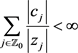, (11)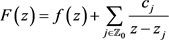, (12)(13)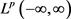的同构映射，且在的任何子域上一致收敛。(14)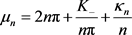, (15)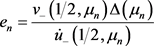, (16), (17)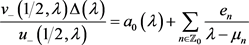. (18)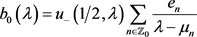, (19)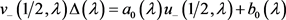. (20)(21)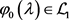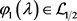，且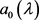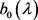在展开式(20)中为唯一的。(22)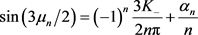,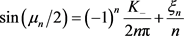, (23)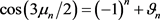,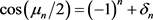,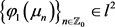. (24)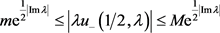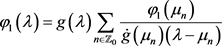, (25)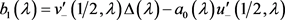, (26)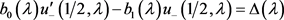. (27)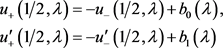(28), (29)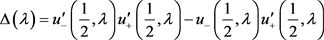(30)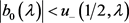，式(30)结合(27)，可知存在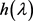满足. (31)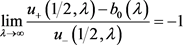,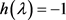，从而可得(28)。定理得证。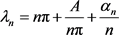(32)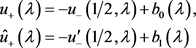(33)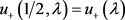,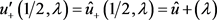.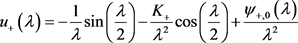,,

NOTES

*通讯作者。

 Hochstadt, H. and Lieberman, B. (1978) An Inverse Sturm-Liouville Problem with Mixed Given Data. SIAM Journal on Applied Mathematics, 34, 676-680.
https://doi.org/10.1137/0134054

 Martinyuk, O. and Pivovarchik, V. (2010) On the Hochstadt-Lieberman Theorem. Inverse Problems, 26, Article ID: 035011, 6 p.
https://doi.org/10.1088/0266-5611/26/3/035011

 Levin, B.J. (1980) Distribution of Zeros of Entire Functions. American Mathematical Society, Providence, RI.

 Marchenko, V. (1986) Sturum-Liouville Operators and Applications. Birkhäuser, Ba-sel.

 Ablowitz, M.J. and Fokas, A.S. (2003) Complex Variables Introduction and Applications. 2nd Edition, Cambridge Univer-sity Press, Cambridge.

 Levin, B. and Yu, I. (1975) Lyubarskii, Interpolation by Entire Functions of Special Classes and Related Expansions in Series of Exponents. Izvestiya Rossiiskoi Akademii Nauk USSR, 39, 657-702. (In Russian)
https://doi.org/10.1070/im1975v009n03abeh001493

 Gesztesy, F. and Simon, B. (2000) Inverse Spectral Analysis with Partial Information on the Potential II: The Case of Discrete Spectrum. Transactions of the American Mathematical Society, 352, 2765-2787.

Top